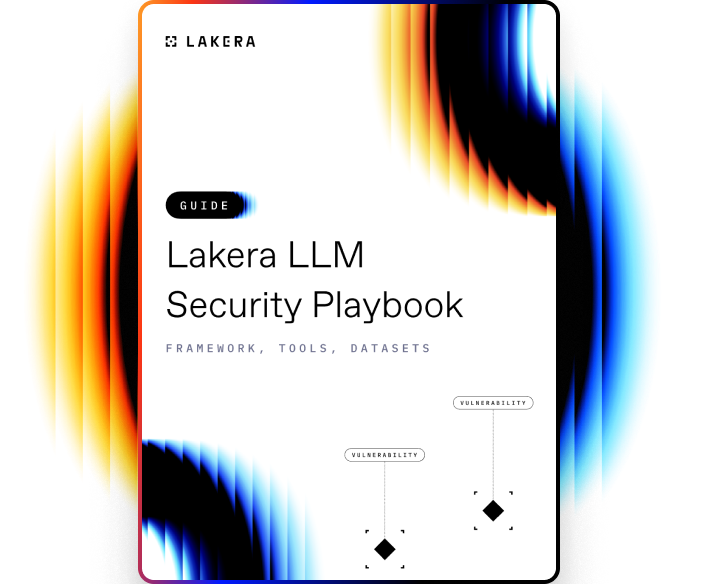VOCABULARY

# K-nearest Neighbour (KNN)

k-nearest neighbour (KNN) is a type of instance-based learning algorithm used in pattern recognition and data mining. It is primarily used for classification and regression. In both cases, the input consists of the k closest training examples in the feature space.

## How KNN works

In the KNN algorithm, the output is a class membership. An object is classified by a plurality vote of its neighbours, with the object being assigned to the class most common among its k-nearest neighbours (k is a positive integer, typically small). If k = 1, then the object is simply assigned to the class of that single nearest neighbour.

In the context of regression, when KNN is used for estimating continuous variables, the output is the property value for the object. This value is the average (or median or mode) of the values of its k-nearest neighbours.

KNN is a type of lazy learning where the function is only approximated locally and all computation is deferred until classification. The KNN algorithm is among the simplest of all machine learning algorithms: both for training and making predictions.

For training, the algorithm requires no additional action other than storing and indexing the dataset. For making predictions, the algorithm calculates the distances between a test point and all training points. The algorithm then selects the k-points closest to the test point. Finally, the algorithm assigns the most common class among those k-points to the test point (or average in case of regression).Learn how to protect against the most common LLM vulnerabilities

Download this guide to delve into the most common LLM security risks and ways to mitigate them.

Related termsActivate
untouchable mode.